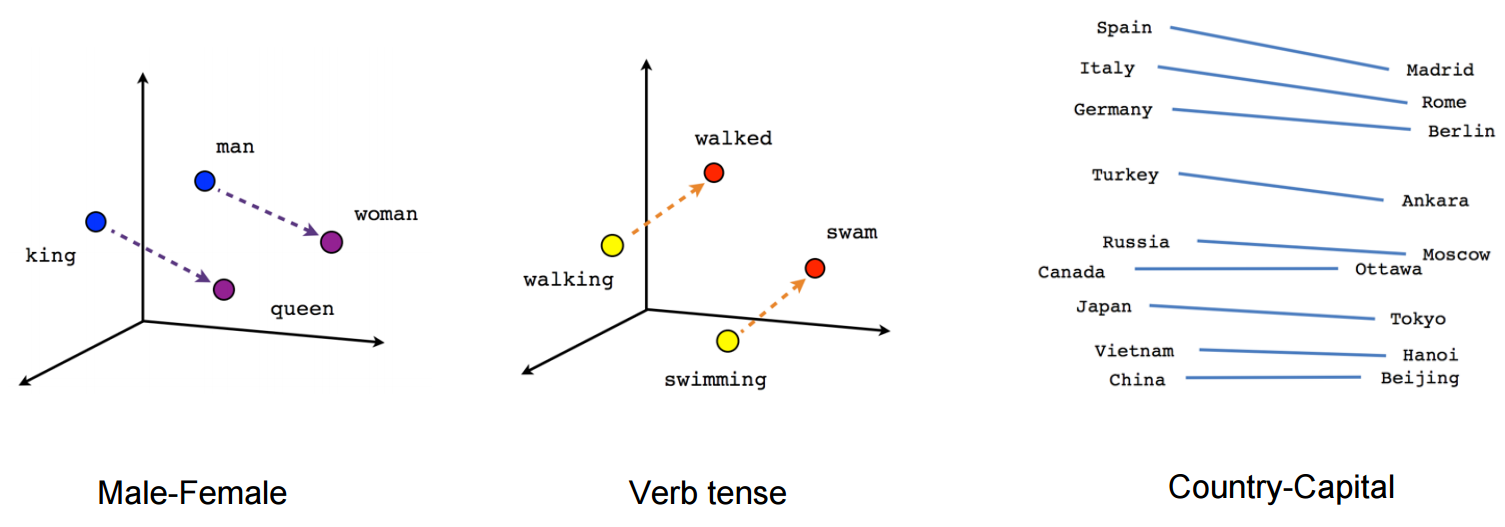2 views

I'm reading the paper below and I have some trouble , understanding the concept of negative sampling.

http://arxiv.org/pdf/1402.3722v1.pdf

machine-learning nlp

closed

+1 vote
by (33.1k points)
edited by

Word2vec is a two-layer neural net that processes text. Word2Vec method is used in Natural Language Processing (NLP) for vectorization of words. Its input is a text corpus and its output is a set of vectors that are feature vectors for words in that corpus. It is used to maximize the similarity between the vectors for words that appear equally likely together in the text, and minimize the similarity of words.The formula for word2vec:

v_c * v_w

-------------------

sum(v_c1 * v_w)

The numerator is the relationship between words c (the context) and w (the target) word. The denominator calculates the similarity of all other contexts c1 and the target word w. Maximizing this ratio ensures words that appear closer together in the text have more similar vectors than words that do not.

Its computations can be quite slow. Negative sampling is one of the ways of solving this problem, just select a couple of contexts c1 at random. For example, if ‘cat’ appears in the context of ‘food’, then the vector of ‘food’ is more similar to the vector of ‘cat’, than the vectors of several other randomly chosen words, instead of all other words in the language. This makes word2vec quite faster to train.

If you want to learn K Means Clustering Algorithm then you can refer to the below video: